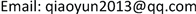﻿ 数理逻辑论证形式有效性算法 The Algorithms about Validity of Argument Form in Logic Mathematician

Pure Mathematics
Vol. 09  No. 03 ( 2019 ), Article ID: 30087 , 6 pages
10.12677/PM.2019.93036

The Algorithms about Validity of Argument Form in Logic Mathematician

Qiaoyun Liu

Qinghai Nationalities University, Xining QinghaiReceived: Apr. 14th, 2019; accepted: Apr. 25th, 2019; published: May 6th, 2019ABSTRACT

It discusses the four methods to judge the validity of argument form in logic mathematician in this paper. In the end it gives the algorithms of judging validity of argument form.

Keywords:Validity of Argument Form, Truth Table, Tautology, Induction RulesCopyright © 2019 by author(s) and Hans Publishers Inc.

This work is licensed under the Creative Commons Attribution International License (CC BY).

http://creativecommons.org/licenses/by/4.0/1. 引言

2. 定义

1) 论证形式定义 

2) 论证形式有效性定义 

3. 论证形式有效性判断方法

1) 用真值表判断

2) 用反证法判断

3) 用重言式判断

4) 用推理规则判断

i) $\text{p}\vee \text{q}$ 前提引入

ii) $\text{p}\to \text{r}$ 前提引入

iii) $\text{q}\to \text{r}$ 前提引入

iv) r (1)(2)(3)构造性两难

v) q 前提引入

vi) $\text{q}\wedge \text{r}$ 合取式

4. 论证形式有效性算法

import java.lang.Math;

public class love {

public static int YH(int p, int q){

if(p == 1 & q == 0){

return 0;}

else{return 1;}}//函数YH为蕴含函数定义；

public static int Y(int array[][],int n,int m){

int cou = 1;int geshu = 0;

int p = 0, q = 0,r = 0, s = 0, t = 0, u = 0;

for(int i = 0; i < n; i ++){

for(int j = 0;j <= m; j ++){

switch(j){

case 0:

p = array[i][j];

break;

case 1:

q = array[i][j];

break;

case 2:

r = array[i][j];

break;

case 3:

s = array[i][j];

break;

case 4:

t = array[i][j];

break;

case 5:

u = array[i][j];//变元不够时可再进行添加；

default:

break;}}

int y = YH(YH(p|q,r)&YH(r,s|t)&YH(t,u)&((~s)&(~u)),((~p)&(q)));//需要验证的论证形式改写用第三种方法写出的语句形式；

array[i] = y;

if(y == 0) cou = 0;}

for(int x = 0; x < array.length / 2; x ++){

int temp = array[x];

array[x] = array[array.length-1-x];

array[array.length-1-x] = temp;}

for(int i = 0; i < array.length; i ++){

if(array[i]==0){

System.out.print(\n此论证形式无效。\n);break;}//只要遇到赋值时结果为0，此语句形式不是重言式，此论证形式无效；

else{geshu ++;}}

if(geshu==n){System.out.print(\n此论证形式有效。\n);}//所有赋值结果都为1时，此语句为重言式，论证形式有效；

return 0;}

public static void main(String args[]){

String str = YH(YH(p|q,r)&YH(r,s|t)&YH(t,u)&((~s)&(~u)),((~p)&(q)))//与int y保持一致；

char[] cha1 = new char[str.length()];

char[] cha2 = new char[str.length()];

for(int i =0; i < str.length(); i ++){

cha1[i] = str.charAt(i);}

int count = 0;

for(int j = 0; j < str.length(); j ++){

if(((int)(cha1[j]) >= 97 & (int)(cha1[j]) <= 122)){

for(int k = j+1; k < str.length(); k ++){

if(cha1[j] == cha1[k]){

cha2[j] = cha1[j];}}}//计算小写字母个数；

else{

if(((int)(cha1[j]) > 122)| ((int)(cha1[j]) < 97)){

cha2[j] = cha1[j];}}}//计算其它字符个数；

for(int i =0; i < cha2.length; i ++){

if((int)(cha2[i]) != 0){

count ++;} }//计算重复的小写字母个数；

count = (int) (str.length()) - count;//计算输入的语句形式有几个变元；

//System.out.print(\n此论证形式变元个数为：+count+\n);此处可输出；

int[][] cha3 = new int[(int)(Math.pow(2,count))][count+1];

for(int i = (int)(Math.pow(2,count))-1; i >= 0; i --){

int s = i;

int t = i;

while(s != 0){

for(int j = 0;j < count;j ++){

cha3[t][j] = s % 2;

s = s / 2;}}}//采用变元赋值时每次十进制多1的方法，将十进制改写成2进制形式给变元赋值；

//System.out.print( +cha3[t][j]);//输出每个变元的赋值；可输出

//System.out.println();}}

for(int j = 0;j < count;j ++){

cha3[j] = 0;}

//System.out.print( +cha3[j]);//输出每个变元的赋值；可输出

System.out.println();

int y= Y(cha3,(int)(Math.pow(2,count)),count+1);} }//调用Y函数计算最后结果。

The Algorithms about Validity of Argument Form in Logic Mathematician[J]. 理论数学, 2019, 09(03): 276-281. https://doi.org/10.12677/PM.2019.93036

1. 1. Hamilton, A.G. Logic for Mathematicians (Revised Edition). 北京: 清华大学出版社, 2003.

2. 2. 王礼萍, 等. 离散数学简明教程[M]. 北京: 清华大学出版社, 2005.